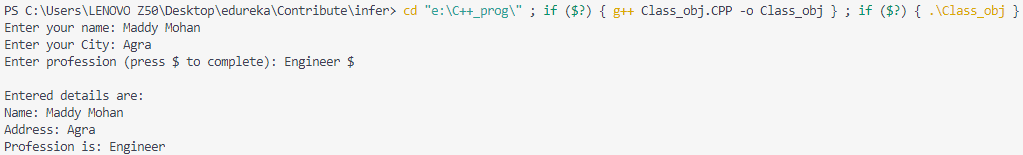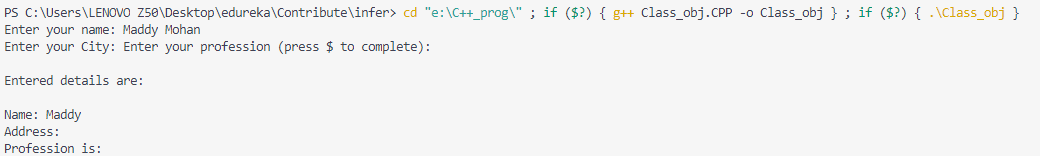# getline (string) in C++

The C++ getline() is a standard library function that is used to read a string or a line from an input stream. It is a part of the <string> header. The getline() function extracts characters from the input stream and appends it to the string object until the delimiting character is encountered. While doing so the previously stored value in the string object str will be replaced by the input string if any.
The getline() function can be represented in two ways:

Syntax:

```istream& getline(istream& is,
string& str, char delim);```

2. Parameters:

• is: It is an object of istream class and tells the function about the stream from where to read the input from.
• str: It is a string object, the input is stored in this object after being read from the stream.
• delim: It is the delimitation character which tells the function to stop reading further input after reaching this character.

Example: To demonstrate the use of delimiter in the getline() function.

## C++

 `#include  ` `#include  ` ` `  `using` `namespace` `std; ` ` `  `//macro definitions ` `#define MAX_NAME_LEN 60  // Maximum len of your name can't be more than 60 ` `#define MAX_ADDRESS_LEN 120  // Maximum len of your address can't be more than 120 ` `#define MAX_ABOUT_LEN 250 // Maximum len of your profession can't be more than 250 ` ` `  `int` `main () { ` `  ``char` `y_name[MAX_NAME_LEN], y_address[MAX_ADDRESS_LEN], about_y[MAX_ABOUT_LEN]; ` ` `  `  ``cout << ``"Enter your name: "``; ` `  ``cin.getline (y_name, MAX_NAME_LEN); ` ` `  `  ``cout << ``"Enter your City: "``; ` `  ``cin.getline (y_address, MAX_ADDRESS_LEN); ` ` `  `  ``cout << ``"Enter your profession (press \$ to complete): "``; ` `  ``cin.getline (about_y, MAX_ABOUT_LEN, ``'\$'``);    ``//\$ is a delimiter  ` ` `  `  ``cout << ``"\nEntered details are:\n"``<<``'\n'``; ` `  ``cout << ``"Name: "` `<< y_name << endl; ` `  ``cout << ``"Address: "` `<< y_address << endl; ` `  ``cout << ``"Profession is: "` `<< about_y << endl; ` `}`

Output:Output

Note: In the above example if the  #define MAX_NAME_LEN  6, So in this case if you cross the defined limit then, in this case, your program will stop execution and exit it’s applicable for every macro that you have used with getline() function. And you’ll get the output as below:

## C++

 `#include  ` `#include  ` ` `  `using` `namespace` `std; ` ` `  `//macro definitions ` `#define MAX_NAME_LEN 60  // Maximum length of your name can't be more than 60 ` `#define MAX_ADDRESS_LEN 120  // Maximum length of your address can't be more than 120 ` `#define MAX_ABOUT_LEN 250 // Maximum length of your profession can't be more than 250 ` ` `  `int` `main () { ` `  ``char` `y_name[MAX_NAME_LEN], y_address[MAX_ADDRESS_LEN], about_y[MAX_ABOUT_LEN]; ` ` `  `  ``cout << ``"Enter your name: "``; ` `  ``cin.getline (y_name, MAX_NAME_LEN); ` ` `  `  ``cout << ``"Enter your City: "``; ` `  ``cin.getline (y_address, MAX_ADDRESS_LEN); ` ` `  `  ``cout << ``"Enter your profession (press \$ to complete): "``; ` `  ``cin.getline (about_y, MAX_ABOUT_LEN, ``'\$'``);    ``//\$ is a delimiter  ` ` `  `  ``cout << ``"\n\nEntered details are:\n\n"``; ` `  ``cout << ``"Name: "` `<< y_name << endl; ` `  ``cout << ``"Address: "` `<< y_address << endl; ` `  ``cout << ``"Profession is: "` `<< about_y << endl; ` `}`

Output:Output_2nd

Here, it is understandable that the length of the name field was more than the defined limit that’s why the program stop execution and exit.

1. Syntax:

`istream& getline (istream& is, string& str);`

2. The second declaration is almost the same as that of the first one. The only difference is, the latter have an delimitation character which is by default newline(\n)character.
Parameters:

• is: It is an object of istream class and tells the function about the stream from where to read the input from.
• str: It is a string object, the input is stored in this object after being read from the stream.

Below program demonstrates the working of the getline() function
Example 1:

## CPP

 `// C++ program to demonstrate getline() function ` ` `  `#include ` `#include ` `using` `namespace` `std; ` ` `  `int` `main() ` `{ ` `    ``string str; ` ` `  `    ``cout << ``"Please enter your name: \n"``; ` `    ``getline(cin, str); ` `    ``cout << ``"Hello, "` `<< str ` `         ``<< ``" welcome to GfG !\n"``; ` ` `  `    ``return` `0; ` `} `

Input:

`Harsh Agarwal`

Output:

`Hello, Harsh Agarwal welcome to GfG!`

Example 2: We can use getline() function to split a sentence on the basis of a character. Let’s look at an example to understand how it can be done.

## CPP

 `// C++ program to understand the use of getline() function ` ` `  `#include ` `using` `namespace` `std; ` ` `  `int` `main() ` `{ ` `    ``string S, T; ` ` `  `    ``getline(cin, S); ` ` `  `    ``stringstream X(S); ` ` `  `    ``while` `(getline(X, T, ``' '``)) { ` `        ``cout << T << endl; ` `    ``} ` ` `  `    ``return` `0; ` `} `

Input:

`Hello, Faisal Al Mamun. Welcome to GfG!`

Output:

```Hello,
Faisal
Al
Mamun.
Welcome
to
GfG!```

Caution :This function considers a new line or (‘\n’) character as the delimitation character and new line character is valid input for this function.
Example of how new line can cause problem is given below:
Example:

## CPP

 `// C++ program to demonstrate ` `// anomaly of delimitation of ` `// getline() function ` `#include ` `#include ` `using` `namespace` `std; ` ` `  `int` `main() ` `{ ` `    ``string name; ` `    ``int` `id; ` ` `  `    ``// Taking id as input ` `    ``cout << ``"Please enter your id: \n"``; ` `    ``cin >> id; ` ` `  `    ``// Takes the empty character as input ` `    ``cout << ``"Please enter your name: \n"``; ` `    ``getline(cin, name); ` ` `  `    ``// Prints id ` `    ``cout << ``"Your id : "` `<< id << ``"\n"``; ` ` `  `    ``// Prints nothing in name field ` `    ``// as "\n" is considered a valid string ` `    ``cout << ``"Hello, "` `<< name ` `         ``<< ``" welcome to GfG !\n"``; ` ` `  `    ``// Again Taking string as input ` `    ``getline(cin, name); ` ` `  `    ``// This actually prints the name ` `    ``cout << ``"Hello, "` `<< name ` `         ``<< ``" welcome to GfG !\n"``; ` ` `  `    ``return` `0; ` `} `

Input:

```7
MOHIT KUMAR```

Output:

```Your id : 7
Hello,  welcome to GfG !
Hello, MOHIT KUMAR welcome to GfG !```

Related Articles:

If you like GeeksforGeeks and would like to contribute, you can also write an article using write.geeksforgeeks.org or mail your article to review-team@geeksforgeeks.org. See your article appearing on the GeeksforGeeks main page and help other Geeks.